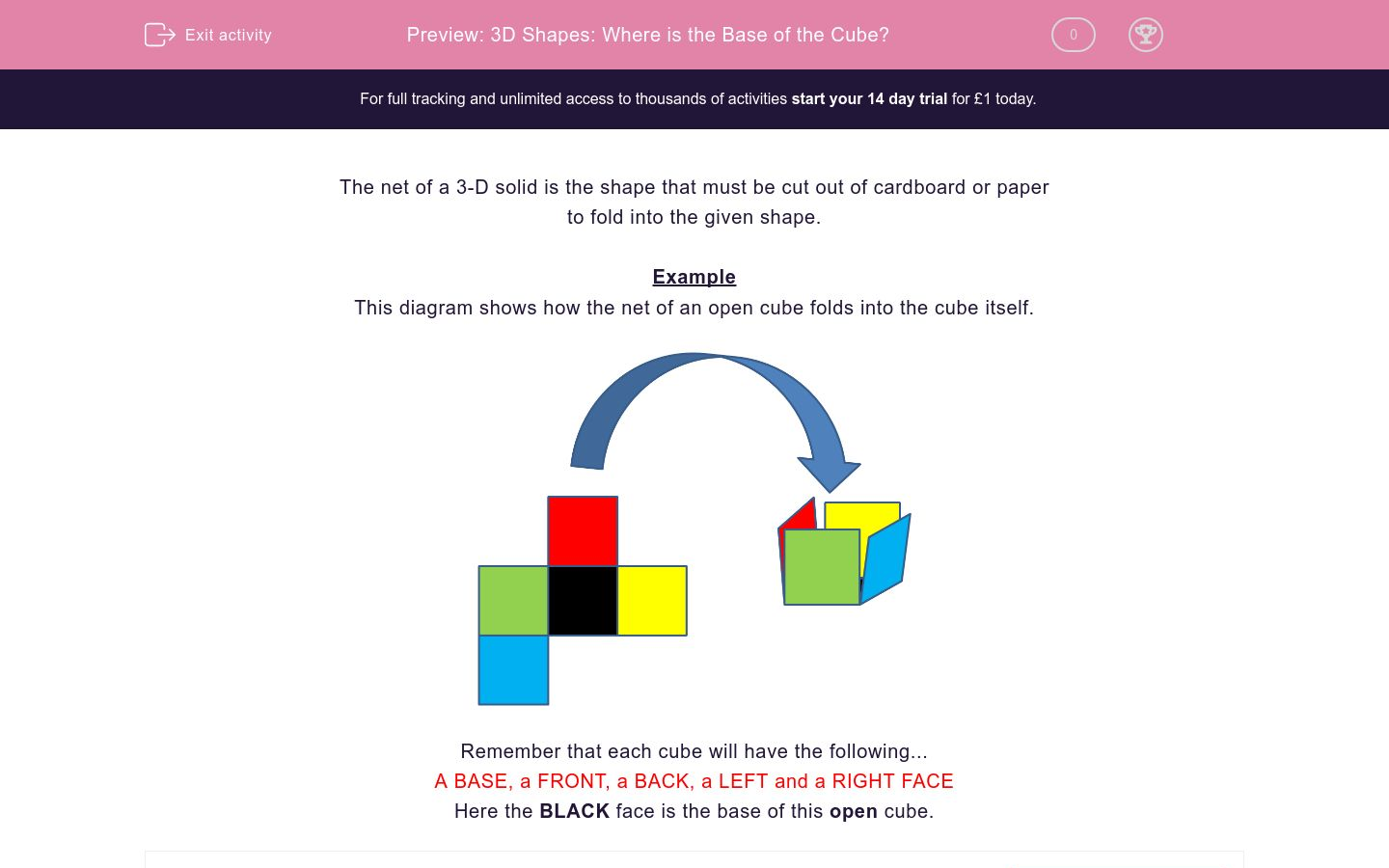# 3D Shapes: Where is the Base of the Cube?

In this worksheet, students identify the bases of open cubes from their nets.Key stage:  KS 2

Curriculum topic:  Maths and Numerical Reasoning

Curriculum subtopic:  3D Shapes

Difficulty level:### QUESTION 1 of 10

The net of a 3-D solid is the shape that must be cut out of cardboard or paper

to fold into the given shape.

Example

This diagram shows how the net of an open cube folds into the cube itself.Remember that each cube will have the following...

A BASE, a FRONT, a BACK, a LEFT and a RIGHT FACE

Here the BLACK face is the base of this open cube.

Here is a net for an open cube.

Which square is the cube's base?A

B

C

D

E

Here is a net for an open cube.

Which square is the cube's base?A

B

C

D

E

Here is a net for an open cube.

Which square is the cube's base?A

B

C

D

E

Here is a net for an open cube.

Which square is the cube's base?A

B

C

D

E

Here is a net for an open cube.

Which square is the cube's base?A

B

C

D

E

Here is a net for an open cube.

Which square is the cube's base?A

B

C

D

E

Here is a net for an open cube.

Which square is the cube's base?A

B

C

D

E

Here is a net for an open cube.

Which square is the cube's base?A

B

C

D

E

Here is a net for an open cube.

Which square is the cube's base?A

B

C

D

E

Here is a net for an open cube.

Which square is the cube's base?A

B

C

D

E

• Question 1

Here is a net for an open cube.

Which square is the cube's base?C
• Question 2

Here is a net for an open cube.

Which square is the cube's base?A
• Question 3

Here is a net for an open cube.

Which square is the cube's base?A
• Question 4

Here is a net for an open cube.

Which square is the cube's base?C
• Question 5

Here is a net for an open cube.

Which square is the cube's base?E
• Question 6

Here is a net for an open cube.

Which square is the cube's base?C
• Question 7

Here is a net for an open cube.

Which square is the cube's base?B
• Question 8

Here is a net for an open cube.

Which square is the cube's base?B
• Question 9

Here is a net for an open cube.

Which square is the cube's base?D
• Question 10

Here is a net for an open cube.

Which square is the cube's base?E
---- OR ----

Sign up for a £1 trial so you can track and measure your child's progress on this activity.

### What is EdPlace?

We're your National Curriculum aligned online education content provider helping each child succeed in English, maths and science from year 1 to GCSE. With an EdPlace account you’ll be able to track and measure progress, helping each child achieve their best. We build confidence and attainment by personalising each child’s learning at a level that suits them.

Get startedStart your £1 trial today.
Subscribe from £10/month.